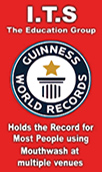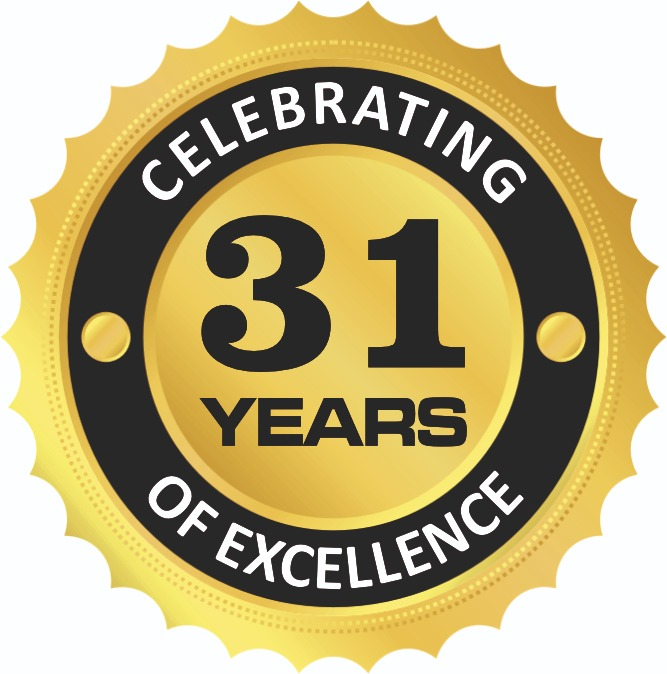Enquiry Now### ITS-UG Campus organized a guest lecture on the topi – “Introduction to Mathematica “for BCA First Year Students on June 1st, 2022

01-06-2022

The objective behind this guest lecture was to expose the students on the topic “Introduction to Mathematica”. The aim of this guest lecture was to provide the knowledge about the Mathematica programming by which we can solve the mathematical question on software. The Resource Person for the guest lecture was renowned teacher and educationist Dr. Deepti Assistant professor from Shivaji College (Delhi University). She discussed about the mathematical language i.e., Mathematica and explained the students that how we can easily find the solution of mathematical problem by using the programming (Mathematica). Overall, the session was highly interactive.